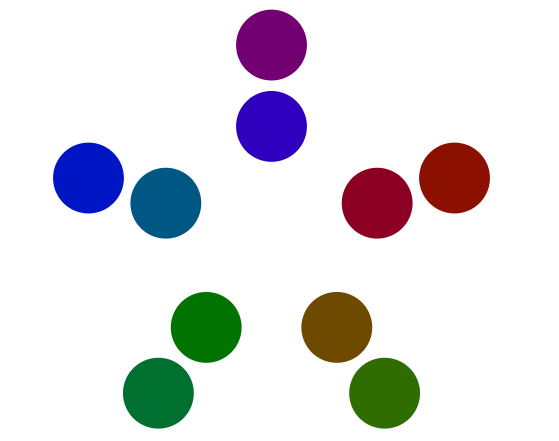### Seeing Numbers Visually

posted Oct 1, 2020, 4:57 PM by Patrick Johnson
 This week we discussed that powerful math learning happens when we think about numbers as symbols while also visualizing and drawing the numbers.Students looked at the drawing below. They were asked what number did each drawing represent  (they visually represent the numbers from 1 to 21) and what did see when they looked at that number.We also discussed how we saw the number (for example 9 is made up of groups of 3). Students were then asked to colour code their math thinking. Many students broke down the number into factors (for example 12 as 3 groups of 4). Others saw shapes in the numbers (for example 16 looks likes 4 squares).We also looked at this cool website that displays numbers visually counting from 1 to 1000 and beyond!(Click the picture below to visit the website)Comments